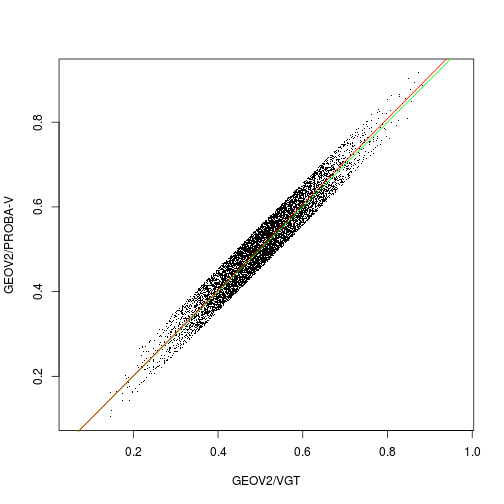# Test slope ==1 for fake GEOV2 data

knitr::opts_chunk$set(fig.path = "figure/FitFig51_")  Generate fake data similar to Fig. 51 FAPAR x <- rnorm(8455,m=0.5,s=0.1) range(x)  ##  0.1450461 0.8833186  According to the reported fit (Fig. 51) y <- 0 + 1.01*x + runif(8455,-0.05,0.05)  Fit a linear model by LS fit <- lm(y ~ x) plot(x,y,pch=".",asp=1,xlab="GEOV2/VGT", ylab="GEOV2/PROBA-V") abline(a=0,b=1,col="green") abline(fit,col="red")sfit <- summary(fit) sfit  ## ## Call: ## lm(formula = y ~ x) ## ## Residuals: ## Min 1Q Median 3Q Max ## -0.049780 -0.024762 -0.000362 0.024741 0.050723 ## ## Coefficients: ## Estimate Std. Error t value Pr(>|t|) ## (Intercept) -0.001383 0.001599 -0.865 0.387 ## x 1.011928 0.003131 323.230 <2e-16 *** ## --- ## Signif. codes: 0 '***' 0.001 '**' 0.01 '*' 0.05 '.' 0.1 ' ' 1 ## ## Residual standard error: 0.02879 on 8453 degrees of freedom ## Multiple R-squared: 0.9251, Adjusted R-squared: 0.9251 ## F-statistic: 1.045e+05 on 1 and 8453 DF, p-value: < 2.2e-16  We thus reject H0: b==0 (there is a linear relationship between x and y) Is the slope b significantly != 1? In other words, test H0: b==1 First, check if 1 is within the confidence interval of b confint(fit)  ## 2.5 % 97.5 % ## (Intercept) -0.004518092 0.001751205 ## x 1.005790611 1.018064393  we see it is not. If we need a test: (hacked from http://stackoverflow.com/questions/33060601/test-if-the-slope-in-simple-linear-regression-equals-to-a-given-constant-in-r) tstats <- (1.0-sfit$coefficients[2,1])/sfit\$coefficients[2,2]
pval<- 2 * pt(abs(tstats), df = df.residual(fit), lower.tail = FALSE)
print(pval)

##  0.0001400195


We thus reject H0: b==1